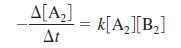# Problem: Consider the hypothetical reaction A 2 (g) + B2 (g) → 2AB (g), where the rate law is:The value of the rate constant at 302°C is 2.45 x 10 -4 L/mol•s, and at 508°C the rate constant is 0.891 L/mol•s. What is the activation energy for this reaction? What is the value of the rate constant for this reaction at 375°C?

###### FREE Expert Solution

Recall: The Arrhenius equation gives us the relationship of the rate constant k with temperature and activation energy Ea. The two-point form of the Arrhenius equation is:We're given:

k1 = 2.45 × 10–4 L/mol•s          T1 = 302 ˚C + 273.15 = 575.15 K

k2 = 0.891 L/mol•s                   T2 = 508°C + 273.15 = 781.15 K

85% (96 ratings)###### Problem Details

Consider the hypothetical reaction A 2 (g) + B2 (g) → 2AB (g), where the rate law is:The value of the rate constant at 302°C is 2.45 x 10 -4 L/mol•s, and at 508°C the rate constant is 0.891 L/mol•s. What is the activation energy for this reaction? What is the value of the rate constant for this reaction at 375°C?

Frequently Asked Questions

What scientific concept do you need to know in order to solve this problem?

Our tutors have indicated that to solve this problem you will need to apply the Arrhenius Equation concept. You can view video lessons to learn Arrhenius Equation. Or if you need more Arrhenius Equation practice, you can also practice Arrhenius Equation practice problems.

What professor is this problem relevant for?

Based on our data, we think this problem is relevant for Professor Anliker's class at IUPUI.

What textbook is this problem found in?

Our data indicates that this problem or a close variation was asked in Chemistry: An Atoms First Approach - Zumdahl Atoms 1st 2nd Edition. You can also practice Chemistry: An Atoms First Approach - Zumdahl Atoms 1st 2nd Edition practice problems.